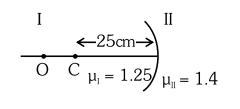# Region I and II are separated by a spherical surface`
Question:

Region I and II are separated by a spherical surface of radius $25 \mathrm{~cm}$. An object is kept in region $\mathrm{I}$ at a distance of $40 \mathrm{~cm}$ from the surface. The distance of the image from the surface is :1. $55.44 \mathrm{~cm}$

2. $9.52 \mathrm{~cm}$

3. $18.23 \mathrm{~cm}$

4. $37.58 \mathrm{~cm}$

Correct Option: , 4

Solution:

$\frac{\mu_{2}}{\mathrm{~V}}-\frac{\mu_{1}}{\mathrm{u}}=\frac{\mu_{2}-\mu_{1}}{\mathrm{R}}$

$\frac{1.4}{v}-\frac{1.25}{-40}=\frac{1.4-1.25}{-25}$

$\frac{1.4}{v}=-\frac{0.15}{25}-\frac{1.25}{40}$

$\mathrm{v}=-37.58 \mathrm{~cm}$

Hence option (4)